You are currently offline. Some features of the site may not work correctly.

# Borsuk–Ulam theorem

Known as: Borsuk Ulam theorem, Borsuk ulam, Borsuk-Ulam theorem
In mathematics, the Borsuk–Ulam theorem (BUT), states that every continuous function from an n-sphere into Euclidean n-space maps some pair of… Expand
Wikipedia

## Papers overview

Semantic Scholar uses AI to extract papers important to this topic.
2017
2017
• 2017
• Corpus ID: 119165965
Barany’s “topological Tverberg conjecture” from 1976 states that any continuous map of an N-simplex $$\Delta _{N}$$ to \(\mathbb… Expand
•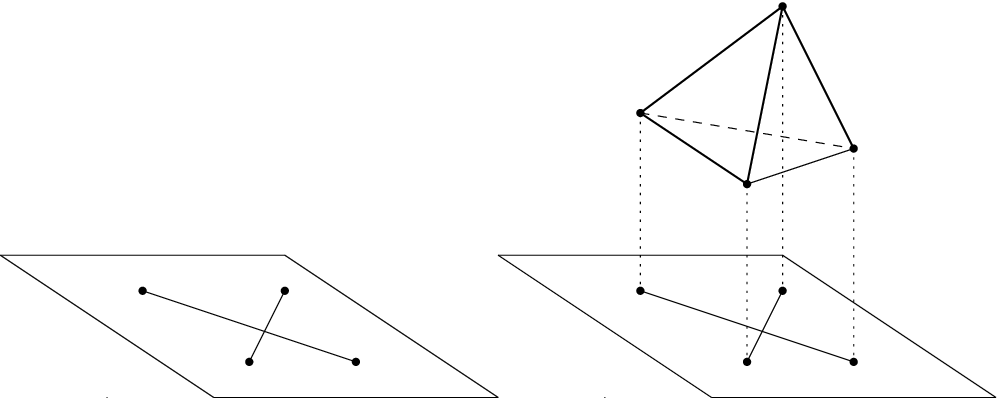•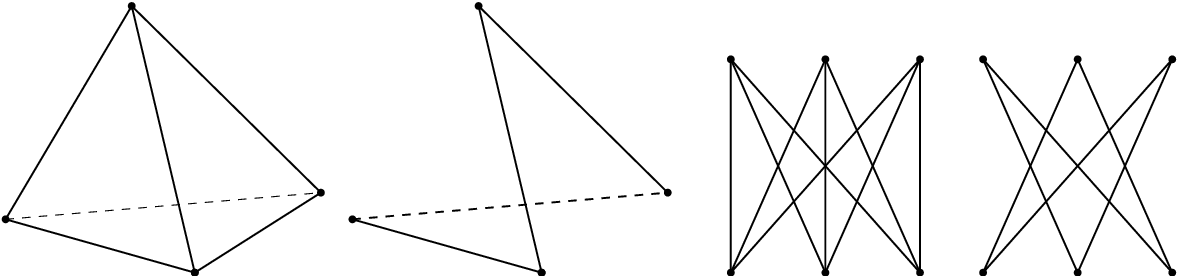•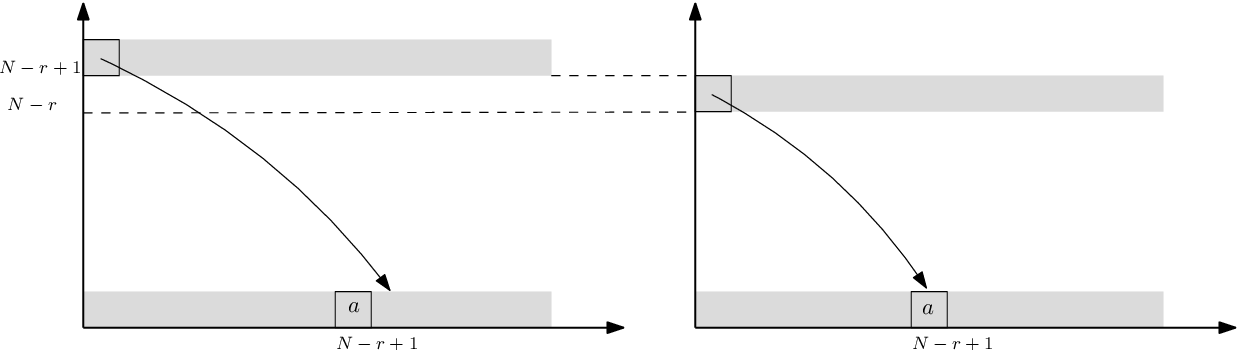•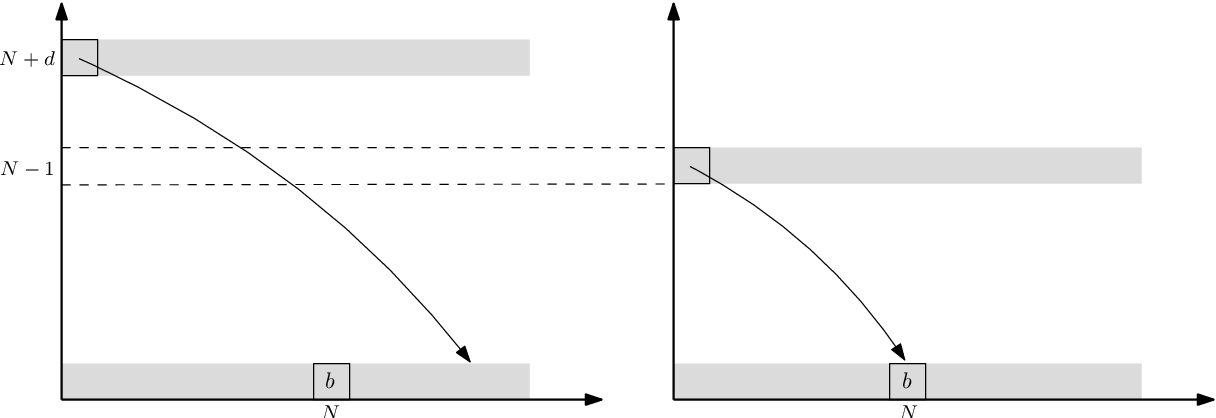2017
2017
• 2017
• Corpus ID: 125683940
The aim of this paper is to compute a simplicial cohomology group of some specific digital images. Then we define ringand algebra… Expand
2016
2016
• 2016
• Corpus ID: 123834194
This paper introduces a region-based extension of the Borsuk-Ulam Theorem (denoted by reBUT). A region is a subset of a surface… Expand
2012
2012
• Formaliz. Math.
• 2012
• Corpus ID: 398502
Summary The Borsuk-Ulam theorem about antipodals is proven, [18, pp. 32-33].
2003
2003
• 2003
• Corpus ID: 34126664
Abstract.Let X, Y be topological spaces and $T : X \rightarrow X$ a free involution. In this context, a question that naturally… Expand
1997
1997
• F. Su
• 1997
• Corpus ID: 11752880
The Borsuk-Ulam theorem and the Brouwer fixed point theorem are well-known theorems of topology with a very similar flavor. Both… Expand
•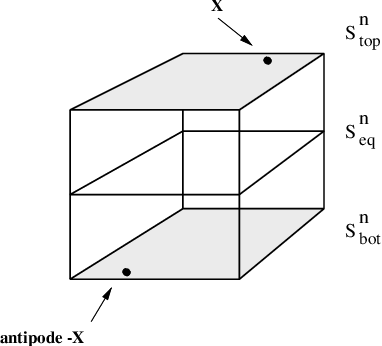•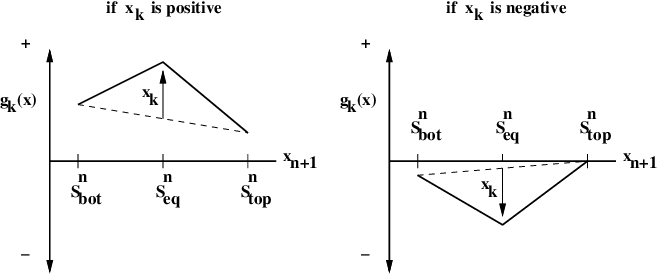1982
1982
Hint. Write every gj as a sum of an even and an odd polynomial. Theorem la is a special case of the well-known Borsuk-Ulam… Expand
Highly Cited
1982
Highly Cited
1982
• 1982
• Corpus ID: 35116552
Abstract : An S to the first power version of the Borsuk-Ulam Theorem is proved for a situation where Fix S to the first power… Expand
1981
1981
ABSTRAcr. Letp: E -B be an n-sphere bundle, q: V-B be an RI-bundle and f: E -V be a fibre preserving map over a paracompact space… Expand
1974
1974
• 1974
• Corpus ID: 54061356
A coincidence theorem generalizing the classical result of Borsuk on maps of Sn into Rn is proved, in which the antipodal map is… Expand